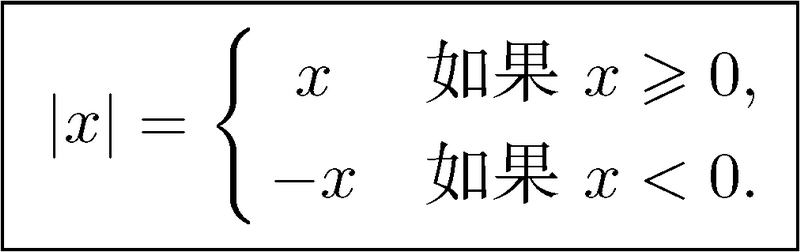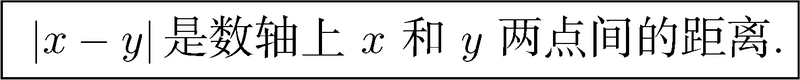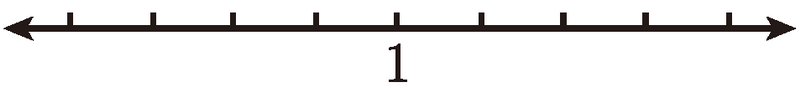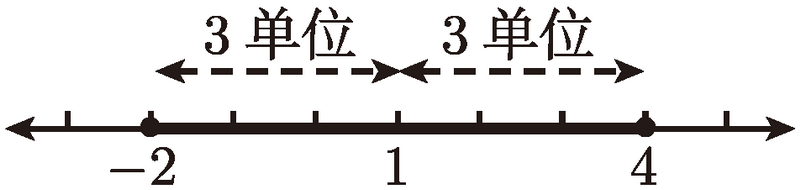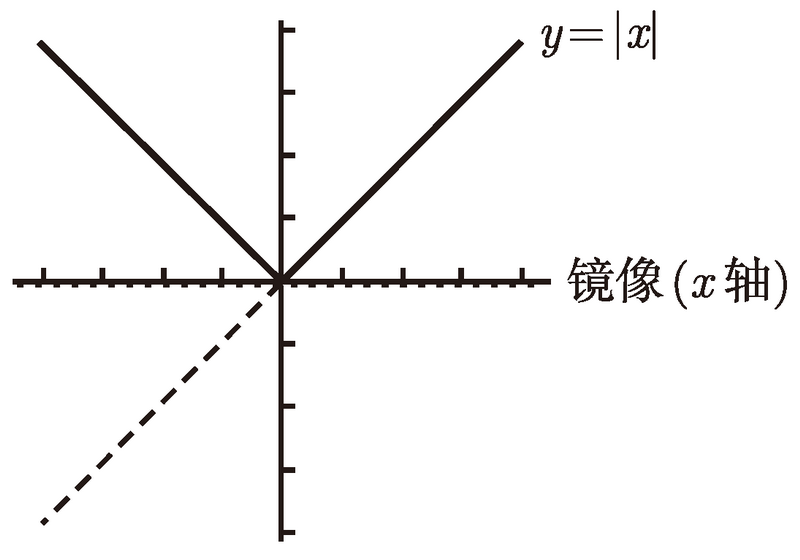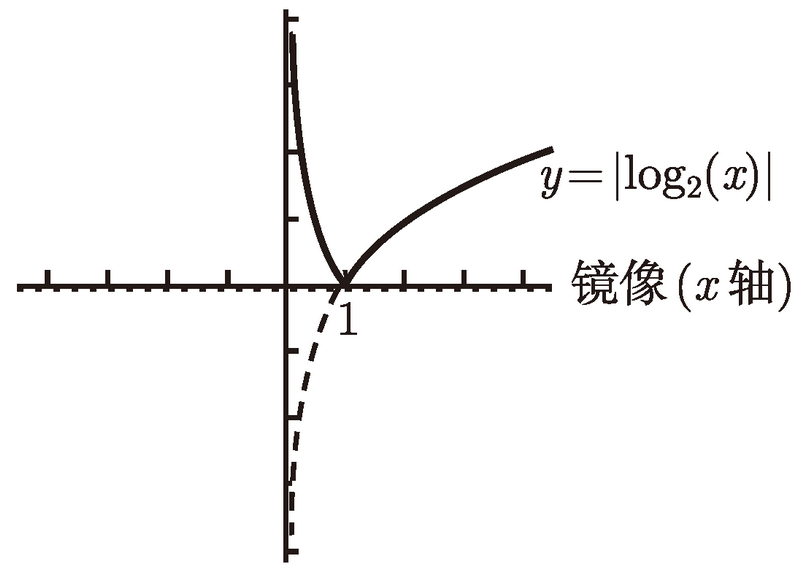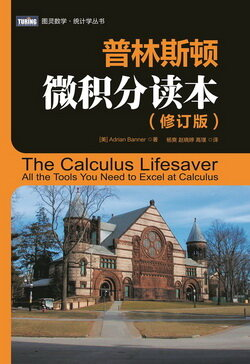# 普林斯顿微积分读本（修订版）(14)：函数、图像和直线 1.6(常见函数及其图像)

(1) 多项式　有许多函数是基于 x 的非负次幂建立起来的. 你可以以 1、xx2x3 等为基本项, 然后用实数同这些基本项做乘法, 最后把有限个这样的项加到一起. 例如, 多项式 f (x) = 5_x_4 -4_x_3 +10 是由 x4 的 5 倍加 x3 的 -4 倍加 10 而形成的. 你可能也想加中间的基本项 x2x , 但由于它们没有出现, 所以我们可以说零倍的 x2 和零倍的 x. 基本项 _xn 的倍数叫作 xn 的系数. 例如, 刚才的多项式 x4x3x2x 和常数项的系数分别为 5、-4、0、0 和 10. (顺便提一下, 为什么会有 x 和 1 的形式? 这两项看上去与其他项不同, 但它们实际上是一样的, 因为 x = x1, 1 = x0.) 最大的幂指数 _n(该项系数不能为零) 叫作多项式的次数. 例如上述多项式的次数为 4, 因为不存在比 4 大的 x 的幂指数. 次数为 n 的多项式的数学通式为

$p(x)=a_nx^n+a_{n-1}x^{n-1}+\cdots+a_2x^2+a_1x+a_0,$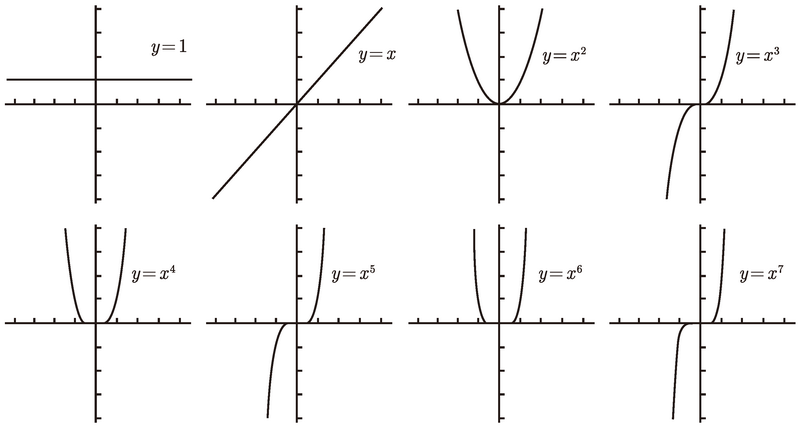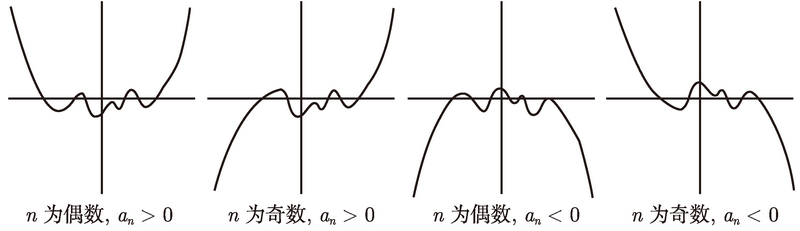$\frac{-b\pm\sqrt{b^2-4ac}}{2a}.$

$x^2-\frac{3}{2}x+5=x^2-\frac{3}{2}x+\frac{9}{16}+5-\frac{9}{16}.$

$x^2-\frac{3}{2}x+5=\biggl(x^2-\frac{3}{2}+\frac{9}{16}\biggr)+5-\frac{9}{16}=\biggl(x-\frac{3}{4}\biggr)^2+5-\frac{9}{16}.$

$2x^2-3x+10=2\biggl(x^2-\frac{3}{2}x+5\biggr)=2\Biggl(\biggl(x-\frac{3}{4}\biggr)^2+\frac{71}{16}\Biggr)=2\biggl(x-\frac{3}{4}\biggr)^2+\frac{71}{8}.$

(2) 有理函数　形如 $\frac{p(x)}{q(x)}$ , 其中 pq 为多项式的函数, 叫作有理函数. 有理函数变化多样, 它的图像根据 pq 两个多项式的变化而变化. 最简单的有理函数是多项式本身, 即 q(x) 为 1 的有理函数. 另一个简单的例子是 1/xn, 其中 n 为正整数. 图 1-17 是一些有理函数的图像.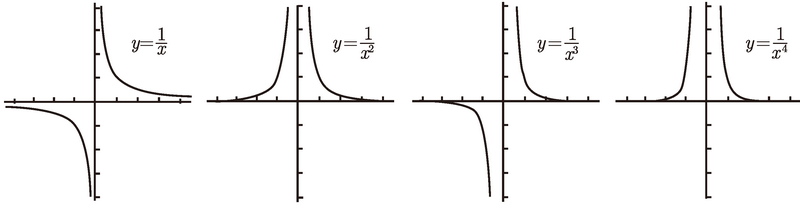(3) 指数函数和对数函数　你需要知道指数函数的图像长什么样. 例如, 图 1-18 是 y = 2x 的图像.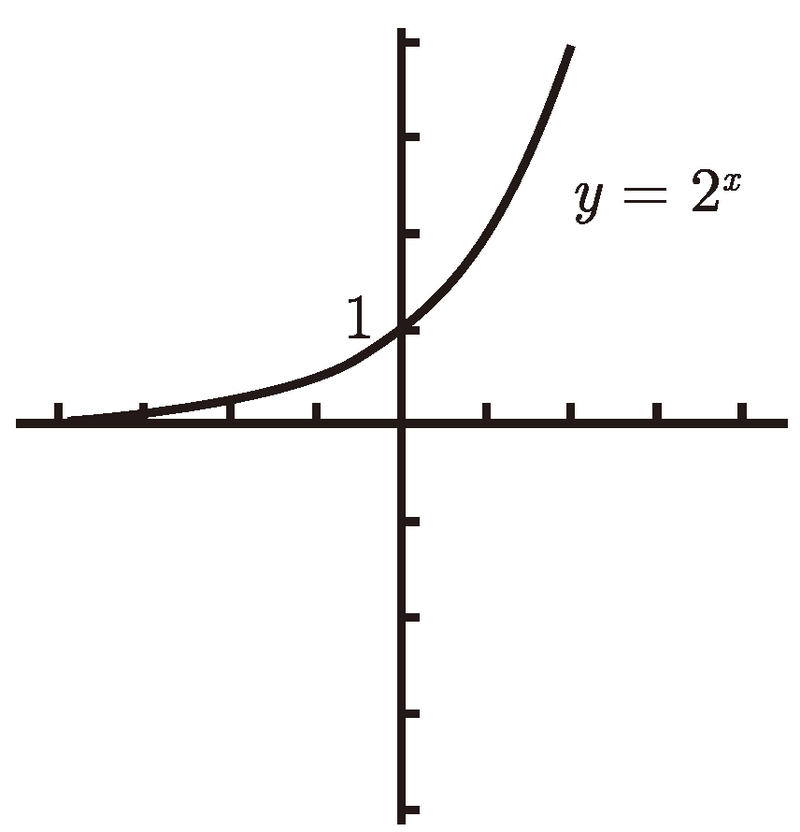y = bx(b > 1) 的图像都与这图类似. 有几点值得注意. 首先, 该函数的定义域为全体实数 ; 其次, y 轴的截距为 1 并且值域为大于零的实数 ; 最后, 左端的水平渐近线为 x 轴. 再强调一下, 该图像非常接近于 x 轴, 但永远不会接触到 x 轴, 无论在你的图形计算器上多么接近. (在第 3 章中, 我们会再次碰到渐近线.) y = 2-x 的图像是 y = 2x 关于 y 轴的对称, 如图 1-19 所示.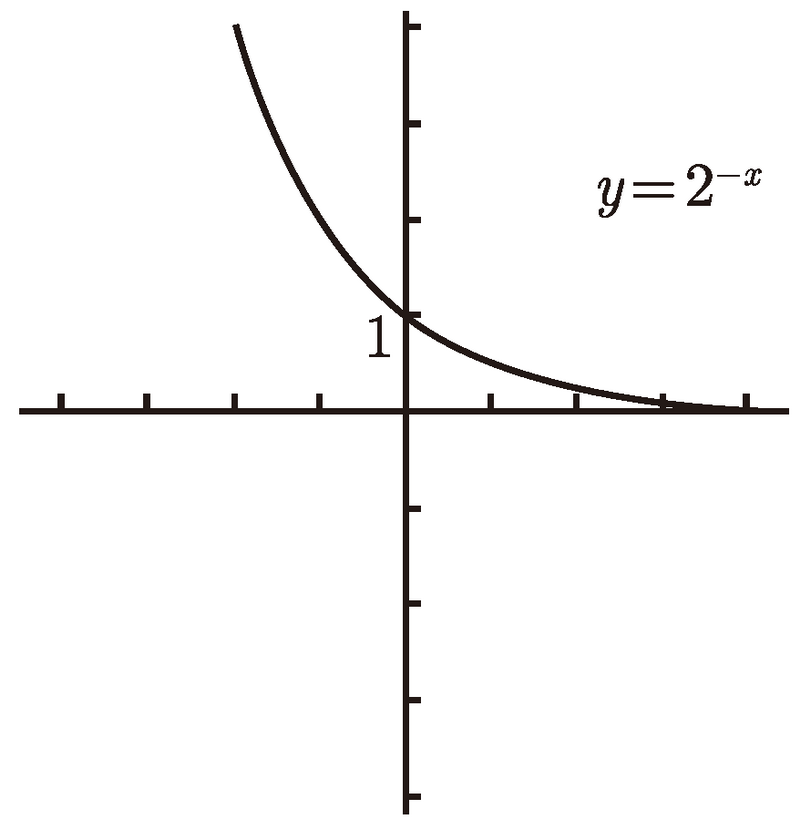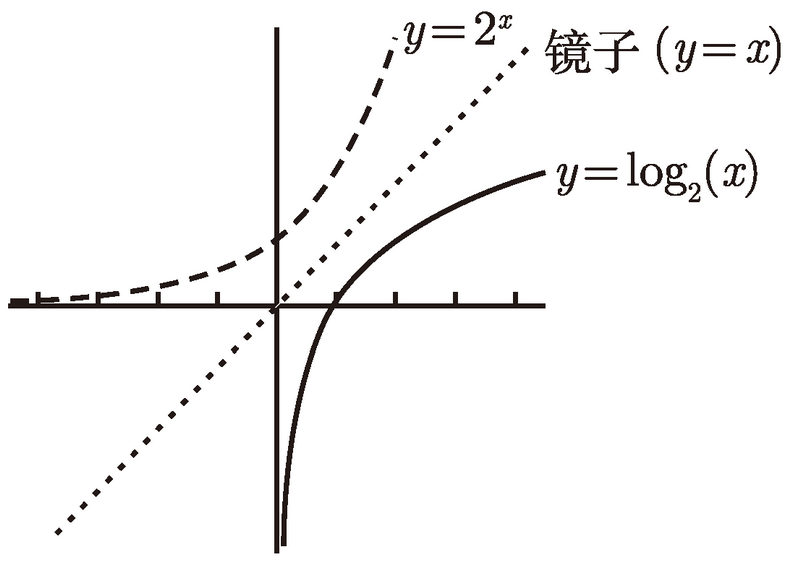(4) 三角函数　三角函数很重要, 所以下一章整章将对其作详细介绍.

(5) 带有绝对值的函数　让我们看一下形如 f (x) = |x| 的绝对值函数. 该函数的定义为：